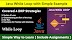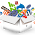FmD4FRX3FmXvDZXvGZT3FRFgNBP1w326w3z1NBMhNV5=

### Video

items# Java While Loop with Simple Example - Simple Way to Learn

Friends, I wanted to teach pattern programs from Java Loops. But most people don't know much about loops. They just remember the loops and after a while forget the steps of the loops in them. You should read this post thoroughly, only then the problems in your while loop will disappear and you will become an expert.

Even if you don't know about this while loop, you can get complete information by reading the post. Here you will learn how to do Dry-Run.

Table of Content

# Java Loop Overview

The concept of loops is very old in programming. If you have learned c and c ++, you have seen different loops in them too. The only difference is that, with the slightest change in the concept of loops, new loops came in the latest programming languages.

In programming, the loops concept is included in the control statement. This includes more if-else statements, break statements, continue statements. The goto statement has been removed from java. Control statements are used to change the flow of a program or to achieve something.

#### What is a loop in java?

Looping in programming languages is a feature that facilitates the execution of a set of instructions/functions repeatedly while some condition evaluates to true.

#### Types of loops in java?

In Java, there are four kinds of loops which are – the for loop, the while loop,  the do-while loop, and the for-each loop. All these four-loop constructs of Java execute a set of repeated statements as long as a specified condition remains true. This particular condition is generally known as loop control.

In this post, you will only see the while loop with a simple example. The next post will contain information about the remaining 3 loops with examples. As well as. You can learn the java-do-while loop by clicking here.

#### 4 Easy Ways to Become an Expert in Java Loops?

1. To know the syntax of loops.
2. Understanding the flow of the loop.
3. To do Dry Run.
4. To practice.
I am going to teach these four options below. Read everything carefully and practice. Comment if you have any problems.

## Java While Loop Overview

The functioning of this loop is also similar to the do-while loop. This while loop repeats a group of statements as long as the condition is true. Once the condition is false, the while loop is terminated.

#### Difference between the do-while loop and the while loop?

In a do-while loop. the statements are executed first and then the condition is tested. On the other hand, in a while loop, the condition is tested first, if it is true, then only the statements are executed.

#### Syntax of a while loop in java

while(condition){
statements;
}

#### What are the 4 steps in the  Java while loop?

Loops have 4 steps, the loops are made up of steps. The structure of the steps in each loop is different. Next, we will see the design of the while loop with an example.

1. Variable Initialization:
From where you want to start your program. Take the datatype and initialize that value.

2. Condition:
You will need to set boundaries for where your program will end. These boundaries are written with the help of the relational operator in JAVA.

3. Statements:
When your loop starts, all the operations you want to do have to be written in the statement block. We will see that in the next example.

4. Increment / Decrement:
The value given to the starting point is taken from the end to the end by the Increment and Decrement operators. Suppose starting number is given as 1 and it has to be increased to 10, then it can be done with the help of the increment operator.

### Java While Loop with Simple Example

I am now going to write a program using a while loop, with the help of which I am going to print the numbers 1 to 5. If you want these examples, there is a download button below, download from there.

class WhileDemo{

public static void main(String[] args) {

int i=1;                                   //Step 1 - Initialization

//Step 2 - Condtion

while(i<=5) {

System.out.println(i);      //Step 3 - Statements
i++;                                    //Step 4 - Increment - Decrement
}
}
}
Output:
1
2
3
4
5
Popular IT Jobs

#### Java While Loop Program Dry Run

If the program in the loop does Dry Run, then the concept of your loop will become more solid. Dry running is considered to be a good habit of programmers.

First I will write the steps and show how the number changed. Then I will add a photo from which you will know how to dry run on the book.

Step 1:
The condition i<=5 is tested. As the condition is true, then the flow of execution will go under the while loop. And the value of i, i.e. 1 so it is displayed the first time. Then the i value is incremented and becomes 2.

Step 2:
The condition i<=5 is tested. As the condition is true, then the flow of execution will go under the while loop. And the value of i, i.e. 2 so it is displayed the second time. Then the i value is incremented and becomes 3.

Step 3:
The condition i<=5 is tested. As the condition is true, then the flow of execution will go under the while loop. And the value of i, i.e. 3 so it is displayed the third time. Then the i value is incremented and becomes 4.

Step 4:
The condition i<=5 is tested. As the condition is true, then the flow of execution will go under the while loop. And the value of i, i.e. 4 so it is displayed the fourth time. Then the i value is incremented and becomes 5.

Step 5:
The condition i<=5 is tested. As the condition is true, then the flow of execution will go under the while loop. And the value of i, i.e. 5 so it is displayed the fifth time. Then the i value is incremented and becomes 6.

Step 6:
Then the condition i<=5 is tested. As the condition is false (value of i, i.e. 6), then the flow of execution will break it.

Program Dry Run:

NOTE -
• If you pass true in the do-while loop, it will be an infinitive do-while loop.
• If you are going to write a single line after a while loop, it works even if no curly brace is given.

#### Java While Loop Examples for Practice

Now your Java While Loop problem will be solved. If there is still any problem, read the post carefully.

Now you want to practice While Loop. Here are some  While Loop examples, you want to solve. Many of these examples also ask the interviewer. Download the following file for its solution.

1. Write a program to print numbers from 1 to 10.
2. Write a program to calculate the sum of the first 20 natural numbers.
3. Write a program to print numbers from 10 to 1.
4. Write a program to find the given no is even or odd.
5. Write a program to print the table of 5.

1.